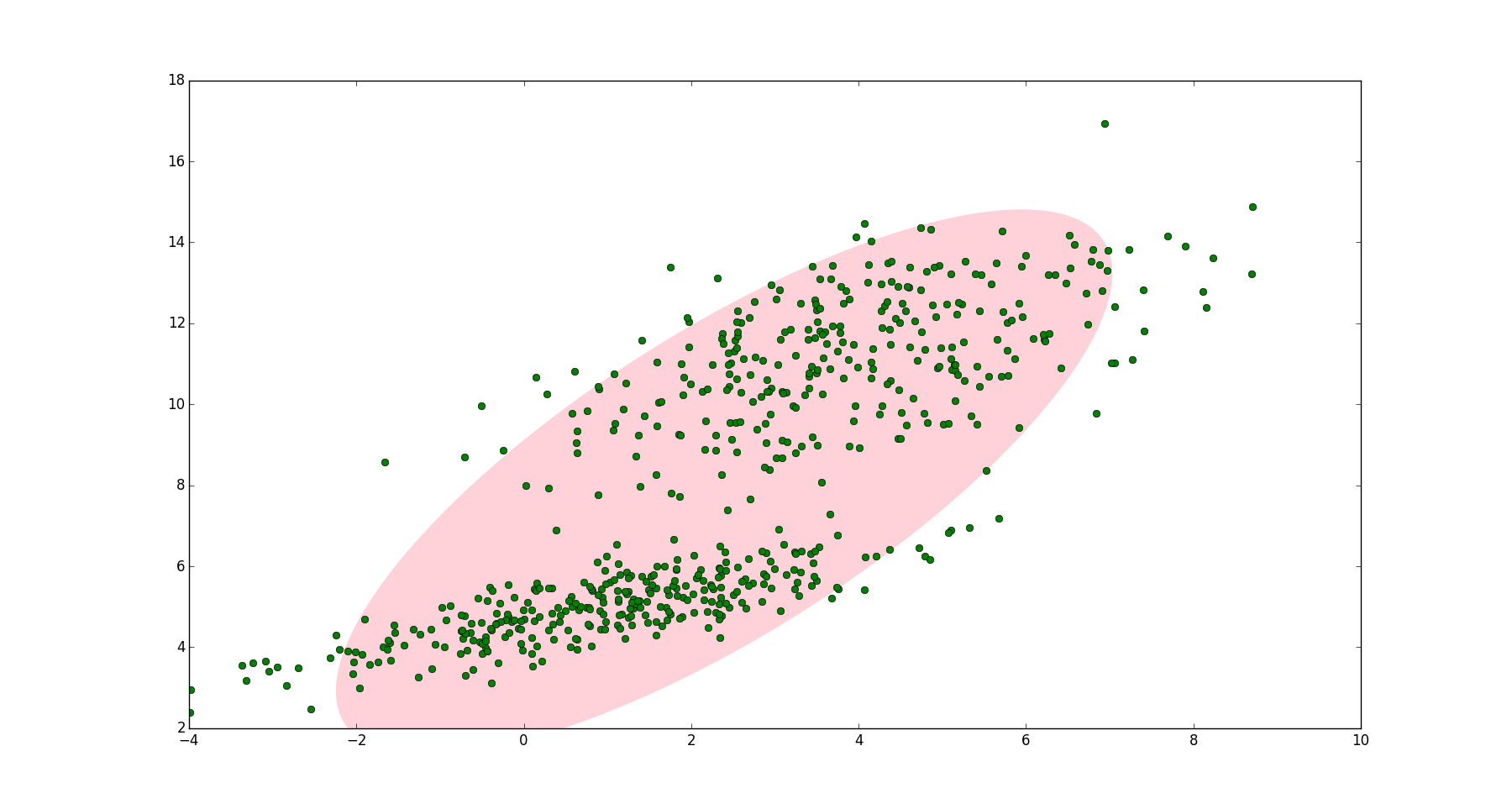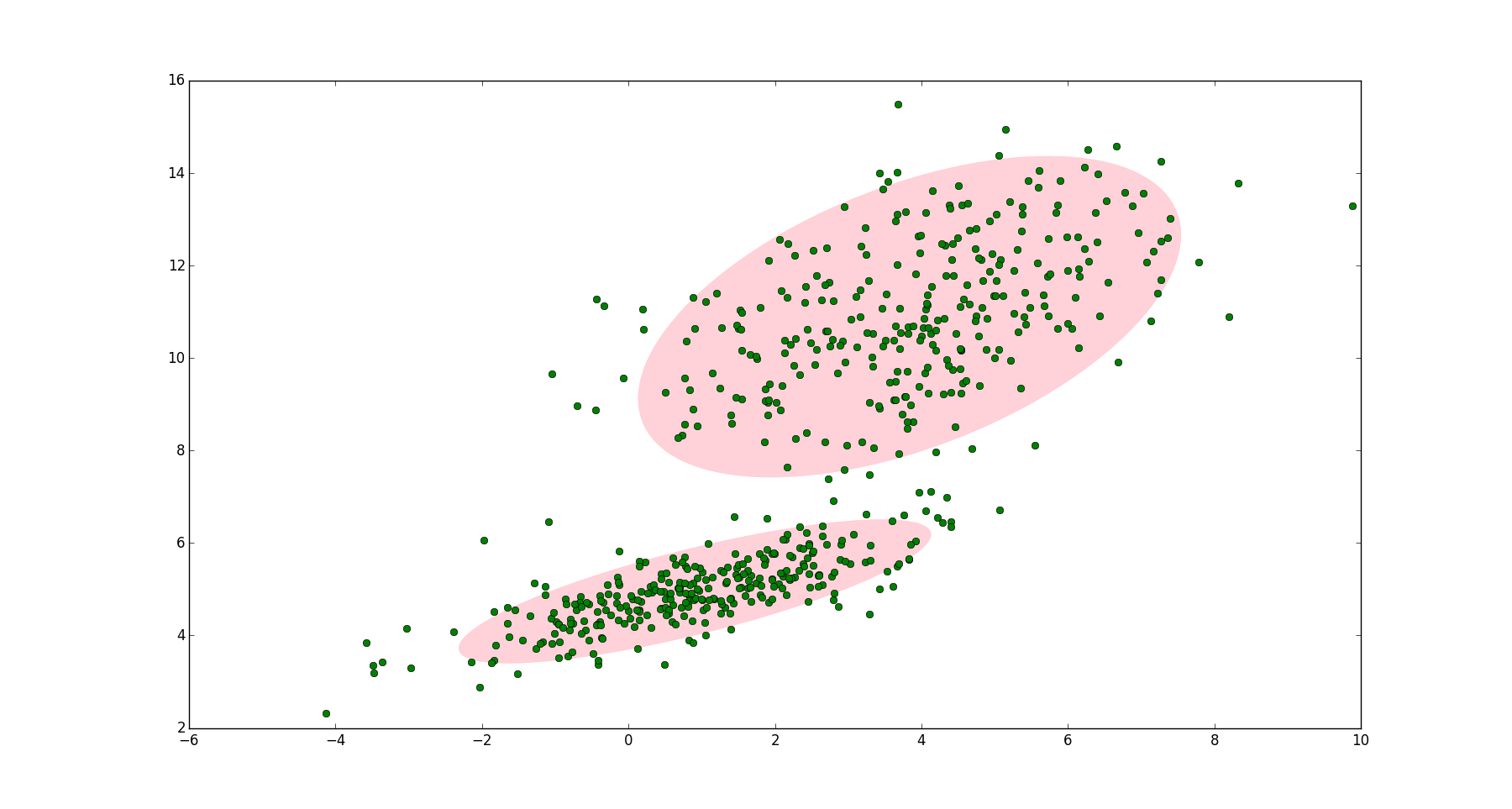# 一个例子# 高斯混合模型（GMM）

$p(\boldsymbol{x}) = \sum_{k=1}^K\pi_k \mathcal{N}(\boldsymbol{x}|\boldsymbol{\mu}_k, \boldsymbol{\Sigma}_k)$

$\sum_{k=1}^K\pi_k = 1$
$0 \leq \pi_k \leq 1$

# GMM的应用

GMM常用于聚类。如果要从 GMM 的分布中随机地取一个点的话，实际上可以分为两步：首先随机地在这 K 个 Component 之中选一个，每个 Component 被选中的概率实际上就是它的系数$\pi_k$ ，选中 Component 之后，再单独地考虑从这个 Component 的分布中选取一个点就可以了──这里已经回到了普通的 Gaussian 分布，转化为已知的问题。

$p(\boldsymbol{x}) =\pi_1 \mathcal{N}(\boldsymbol{x}|\boldsymbol{\mu}_1, \boldsymbol{\Sigma}_1) + \pi_2 \mathcal{N}(\boldsymbol{x}|\boldsymbol{\mu}_2, \boldsymbol{\Sigma}_2)$

# GMM参数估计过程

## GMM的贝叶斯理解

$p(\boldsymbol{x}) = \sum_{k=1}^K\pi_k \mathcal{N}(\boldsymbol{x}|\boldsymbol{\mu}_k, \boldsymbol{\Sigma}_k) \tag{1}$

$z_k \in \{0,1\}$
$\sum_K z_k = 1$

$z_k=1$的概率就是$\pi_k$，假设$z_k$之间是独立同分布的（iid），我们可以写出$\boldsymbol{z}$的联合概率分布形式，就是连乘：

$p(\boldsymbol{z}) = p(z_1) p(z_2)...p(z_K) = \prod_{k=1}^K \pi_k^{z_k} \tag{2}$

$p(\boldsymbol{x}|z_k = 1) = \mathcal{N}(\boldsymbol{x}|\boldsymbol{\mu}_k, \boldsymbol{\Sigma}_k)$

$p(\boldsymbol{x}| \boldsymbol{z}) = \prod_{k=1}^K \mathcal{N}(\boldsymbol{x}|\boldsymbol{\mu}_k, \boldsymbol{\Sigma}_k)^{z_k} \tag{3}$

\begin{aligned} p(\boldsymbol{x}) &= \sum_{\boldsymbol{z}} p(\boldsymbol{z}) p(\boldsymbol{x}| \boldsymbol{z}) \\ &= \sum_{\boldsymbol{z}} \left(\prod_{k=1}^K \pi_k^{z_k} \mathcal{N}(\boldsymbol{x}|\boldsymbol{\mu}_k, \boldsymbol{\Sigma}_k)^{z_k} \right ) \\ &= \sum_{k=1}^K \pi_k \mathcal{N}(\boldsymbol{x}|\boldsymbol{\mu}_k, \boldsymbol{\Sigma}_k) \end{aligned} \tag{4}

（注：上式第二个等号，对$\boldsymbol{z}$求和，实际上就是$\sum_{k=1}^K$。又因为对某个$k$，只要$i \ne k$，则有$z_i = 0$，所以$z_k=0$的项为1，可省略，最终得到第三个等号）

\begin{aligned} \gamma(z_k) &= p(z_k = 1| \boldsymbol{x}) \\ &= \frac{p(z_k = 1) p(\boldsymbol{x}|z_k = 1)}{p(\boldsymbol{x}, z_k = 1)} \\ &= \frac{p(z_k = 1) p(\boldsymbol{x}|z_k = 1)}{\sum_{j=1}^K p(z_j = 1) p(\boldsymbol{x}|z_j = 1)} \text{ (全概率公式)}\\ &= \frac{\pi_k \mathcal{N}(\boldsymbol{x|\boldsymbol{\mu}_k, \boldsymbol{\Sigma}_k})}{\sum_{j=1}^K \pi_j \mathcal{N}(\boldsymbol{x|\boldsymbol{\mu}_j, \boldsymbol{\Sigma}_j})} \text{ (结合(3)、(4))} \end{aligned} \tag{5}

（第2行，贝叶斯定理。关于这一行的分母，很多人有疑问，应该是$p(\boldsymbol{x}, z_k = 1)$还是$p(\boldsymbol{x})$，按照正常写法，应该是$p(\boldsymbol{x})$。但是为了强调$z_k$的取值，有的书会写成$p(\boldsymbol{x}, z_k = 1)$，比如李航的《统计学习方法》，这里就约定$p(\boldsymbol{x})$$p(\boldsymbol{x}, z_k = 1)$是等同的）

## EM算法估计GMM参数

EM算法（Expectation-Maximization algorithm）分两步，第一步先求出要估计参数的粗略值，第二步使用第一步的值最大化似然函数。因此要先求出GMM的似然函数。

$p(\boldsymbol{x}|\boldsymbol{\pi}, \boldsymbol{\mu}, \boldsymbol{\Sigma}) = \sum_{k=1}^K\pi_k \mathcal{N}(\boldsymbol{x}|\boldsymbol{\mu}_k, \boldsymbol{\Sigma}_k) \tag{6}$

$0 = -\sum_{n=1}^N \frac{\pi_k \mathcal{N}(\boldsymbol{x}_n | \boldsymbol{\mu}_k, \boldsymbol{\Sigma}_k)}{\sum_j \pi_j \mathcal{N}(\boldsymbol{x}_n|\boldsymbol{\mu}_j, \boldsymbol{\Sigma}_j)} \boldsymbol{\Sigma}_k^{-1} (\boldsymbol{x}_n - \boldsymbol{\mu}_k) \tag{7}$

$\boldsymbol{\mu}_k = \frac{1}{N_k}\sum_{n=1}^N \gamma(z_{nk}) \boldsymbol{x}_n \tag{8}$

$N_k = \sum_{n=1}^N \gamma(z_{nk}) \tag{9}$

(8)式和(9)式中，$N$表示点的数量。$\gamma(z_{nk})$表示点$n$$\boldsymbol{x}_n$）属于聚类$k$的后验概率。$N_k$可以表示属于第$k$个聚类的点的数量。那么$\boldsymbol{\mu}_k$表示所有点的加权平均，每个点的权值是$\sum_{n=1}^N \gamma(z_{nk})$，跟第$k$个聚类有关。

$\boldsymbol{\Sigma}_k = \frac{1}{N_k} \sum_{n=1}^N \gamma(z_{nk}) (x_n - \boldsymbol{\mu}_k)(x_n - \boldsymbol{\mu}_k)^T \tag{10}$

$\ln p(\boldsymbol{x}|\boldsymbol{\pi}, \boldsymbol{\mu}, \boldsymbol{\Sigma}) + \lambda(\sum_{k=1}^K \pi_k -1)$

$0 = \sum_{n=1}^N \frac{\mathcal{N}(\boldsymbol{x}_n | \boldsymbol{\mu}_k, \boldsymbol{\Sigma}_k)}{\sum_j \pi_j \mathcal{N}(\boldsymbol{x}_n|\boldsymbol{\mu}_j, \boldsymbol{\Sigma}_j)} + \lambda \tag{11}$

$0 = \sum_{n=1}^N \frac{\pi_k \mathcal{N}(\boldsymbol{x}_n | \boldsymbol{\mu}_k, \boldsymbol{\Sigma}_k)}{\sum_j \pi_j \mathcal{N}(\boldsymbol{x}_n|\boldsymbol{\mu}_j, \boldsymbol{\Sigma}_j)} + \lambda \pi_k \tag{11.1}$

$0 = N_k + \lambda \pi_k \tag{11.2}$

$0 = \sum_{k=1}^K N_k + \lambda \sum_{k=1}^K \pi_k \tag{11.3}$

$0 = N + \lambda \tag{11.4}$

$\pi_k = \frac{N_k}{N} \tag{12}$

EM算法估计GMM参数即最大化(8)，(10)和(12)。需要用到(5)，(8)，(10)和(12)四个公式。我们先指定$\boldsymbol{\pi}$$\boldsymbol{\mu}$$\boldsymbol{\Sigma}$的初始值，带入(5)中计算出$\gamma(z_{nk})$，然后再将$\gamma(z_{nk})$带入(8)，(10)和(12)，求得$\pi_k$$\boldsymbol{\mu}_k$$\boldsymbol{\Sigma}_k$；接着用求得的$\pi_k$$\boldsymbol{\mu}_k$$\boldsymbol{\Sigma}_k$再带入(5)得到新的$\gamma(z_{nk})$，再将更新后的$\gamma(z_{nk})$带入(8)，(10)和(12)，如此往复，直到算法收敛。

## EM算法

1. 定义分量数目$K$，对每个分量$k$设置$\pi_k$$\boldsymbol{\mu}_k$$\boldsymbol{\Sigma}_k$的初始值，然后计算(6)式的对数似然函数。
2. E step
根据当前的$\pi_k$$\boldsymbol{\mu}_k$$\boldsymbol{\Sigma}_k$计算后验概率$\gamma(z_{nk})$
$\gamma(z_{nk}) = \frac{\pi_k\mathcal{N}(\boldsymbol{x}_n| \boldsymbol{\mu}_n, \boldsymbol{\Sigma}_n)}{\sum_{j=1}^K \pi_j \mathcal{N}(\boldsymbol{x}_n| \boldsymbol{\mu}_j, \boldsymbol{\Sigma}_j)}$
3. M step
根据E step中计算的$\gamma(z_{nk})$再计算新的$\pi_k$$\boldsymbol{\mu}_k$$\boldsymbol{\Sigma}_k$
\begin{aligned} \boldsymbol{\mu}_k^{new} &= \frac{1}{N_k} \sum_{n=1}^N \gamma(z_{nk}) \boldsymbol{x}_n \\ \boldsymbol{\Sigma}_k^{new} &= \frac{1}{N_k} \sum_{n=1}^N \gamma(z_{nk}) (\boldsymbol{x}_n - \boldsymbol{\mu}_k^{new}) (\boldsymbol{x}_n - \boldsymbol{\mu}_k^{new})^T \\ \pi_k^{new} &= \frac{N_k}{N} \end{aligned}
其中：
$N_k = \sum_{n=1}^N \gamma(z_{nk})$
4. 计算(6)式的对数似然函数
$\ln p(\boldsymbol{x}|\boldsymbol{\pi}, \boldsymbol{\mu}, \boldsymbol{\Sigma}) = \sum_{n=1}^N \ln \left\{\sum_{k=1}^K \pi_k \mathcal{N}(\boldsymbol{x}_k| \boldsymbol{\mu}_k, \boldsymbol{\Sigma}_k)\right\}$
5. 检查参数是否收敛或对数似然函数是否收敛，若不收敛，则返回第2步。

# Reference

1. 漫谈 Clustering (3): Gaussian Mixture Model
2. Draw Gaussian distribution ellipse
3. Pang-Ning Tan 等, 数据挖掘导论（英文版）, 机械工业出版社, 2010
4. Christopher M. Bishop etc., Pattern Recognition and Machine Learning, Springer, 2006
5. 李航, 统计学习方法, 清华大学出版社, 2012

03-187万+

#### 高斯混合模型的终极理解

07-147万+

#### 详解EM算法与混合高斯模型(Gaussian mixture model, GMM)06-191万+

#### 机器学习（十四）-GMM混合高斯模型(Gaussian mixture model)算法及Python实例

11-1912万+

#### GMM的EM算法实现

02-145万+

#### 高斯混合模型（GMM）介绍以及学习笔记

07-161万+

#### 高斯混合模型(GMM)和EM算法

01-034093

#### 高斯混合模型（GMM）和EM算法

12-10218

#### 高斯混合模型（GMM）

03-20352

#### 高斯混合模型GMM聚类的步骤和推导

06-28398

#### EM算法估计混合高斯模型(GMM)

03-158万+

#### 一个HashMap跟面试官扯了半个小时

09-0420万+

#### 爬虫小程序 - 爬取王者荣耀全皮肤

04-101万+

#### EM算法--二维高斯混合模型(GMM)

04-096万+

#### 代码注释如此沙雕，会玩还是你们程序员！

05-288万+

#### GMM-HMM语音识别模型 原理篇

01-235663

#### 高斯混合模型（GMM）及其求解（期望最大化（EM）算法）©️2020 CSDN 皮肤主题: 大白 设计师: CSDN官方博客点击重新获取扫码支付1.余额是钱包充值的虚拟货币，按照1:1的比例进行支付金额的抵扣。
2.余额无法直接购买下载，可以购买VIP、C币套餐、付费专栏及课程。余额充值